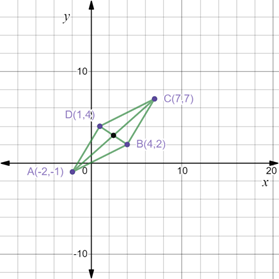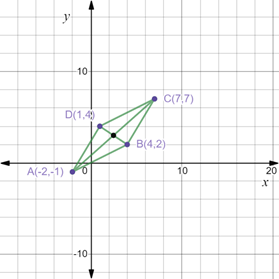# To graph : The parallelogram with the vertices A ( − 2 , − 1 ) , B ( 4 , 2 ) , C ( 7 , 7 ) and D ( 1 , 4 ) .### Precalculus: Mathematics for Calcu...

6th Edition
Stewart + 5 others
Publisher: Cengage Learning
ISBN: 9780840068071### Precalculus: Mathematics for Calcu...

6th Edition
Stewart + 5 others
Publisher: Cengage Learning
ISBN: 9780840068071

#### Solutions

Chapter 1.8, Problem 47E

(a)

To determine

## To graph: The parallelogram with the vertices A(−2,−1),B(4,2),C(7,7) and D(1,4) .

Expert Solution

### Explanation of Solution

Given information:

The vertices of the parallelogram A(2,1),B(4,2),C(7,7) and D(1,4) .

Graph:

The parallelogram with vertices A(2,1),B(4,2),C(7,7) and D(1,4) can be sketched by plotting the given points on a coordinate plane as,Interpretation:

A parallelogram is a convex polygon with two pairs of parallel lines, i.e. its opposite sides are parallel and also they are of equal lengths.

A parallelogram is a quadrilateral whose diagonals bisect each other at the same point.

Opposite angles of a parallelogram are equal i.e. A=C and B=D .

The given vertices of the parallelogram satisfy all the conditions in the plotted figure.

(b)

To determine

### To calculate: The mid-points of the diagonal of the parallelogram ABCD.

Expert Solution

The coordinates of mid-point of parallelogram ABCD is (52,3) .

### Explanation of Solution

Given information:

The vertices of the parallelogram A(2,1),B(4,2),C(7,7) and D(1,4) .

Formula used:

Mid-point formula between two points X(a1,b1) and Y(a2,b2) is mathematically expressed as,

(a1+a22,b1+b22)

Calculation:

Consider the provided vertices of the parallelogram A(2,1),B(4,2),C(7,7) and D(1,4) .

By plotting the given points on the coordinate plane, we get the following figure,Recall that the mid-point formula between two points X(a1,b1) and Y(a2,b2) is mathematically expressed as,

(a1+a22,b1+b22)

So, midpoint of AC is calculated as,

(2+72,1+72)=(52,62)=(52,3)

Now, midpoint of BD is calculated as,

(1+42,4+22)=(52,62)=(52,3)

Thus, the coordinates of mid-point of parallelogram ABCD is (52,3) .

(c)

To determine

Expert Solution

### Explanation of Solution

Given information:

The vertices of the parallelogram A(2,1),B(4,2),C(7,7) and D(1,4) .

Formula used:

If two diagonals are intersected by each other at the same point then the diagonals bisect each other at that point.

Proof:

Consider the given vertices of the parallelogram ABCD A(2,1),B(4,2),C(7,7) and D(1,4) .

From part (b), it is observed that the midpoint of the diagonals of the parallelogram coincide each other.

Recall if two diagonals are intersected by each other at the same point then the diagonals bisect each other at that point.

Thus, it is proved that the diagonals of the parallelogram ABCD bisects each other at the point (52,3) .

### Have a homework question?

Subscribe to bartleby learn! Ask subject matter experts 30 homework questions each month. Plus, you’ll have access to millions of step-by-step textbook answers!